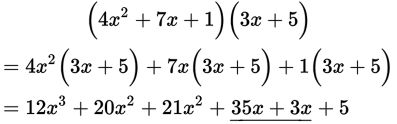# SAT Math Multiple Choice Question 353: Answer and Explanation

### Test Information

Question: 353

8. If 4x2 + 7x + 1 is multiplied by 3x + 5, what is the coefficient of x in the resulting polynomial?

• A. 3
• B. 12
• C. 35
• D. 38

Explanation:

D

Difficulty: Medium

Category: Passport to Advanced Math / Exponents

Strategic Advice: When multiplying polynomials, carefully multiply each term in the first factor by each term in the second factor. This question doesn't ask for the entire product, so check to make sure you answered the right question (the coefficient of x).

Getting to the Answer: After performing the initial multiplication, look for the x-terms and add their coefficients. To save time, you do not need to simplify the other terms in the expression.The coefficient of x is 35 + 3 = 38.